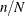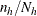#### Unrestricted Random Sampling

The method of unrestricted random sampling (METHOD=URS) selects units with equal probability and with replacement. Because units are selected with replacement, a unit can be selected for the sample more than once. The expected number of hits (selections) for each unit equalswhen sampling without stratification. For stratified sampling, the expected number of hits for a unit in stratum h equals. Note that the expected number of hits exceeds 1 when the sample size n is greater than the population size N.

For unrestricted random sampling, by default, the output data set contains a single copy of each unit selected, even when a unit is selected more than once, and the variable `NumberHits` records the number of hits (selections) for each unit. If you specify the OUTHITS option, the output data set contains m copies of a sampling unit for which `NumberHits` equals m. For example, with the OUTHITS option a unit that is selected three times is represented by three copies in the output data set. For information about the contents of the output data set, see the section Sample Output Data Set.